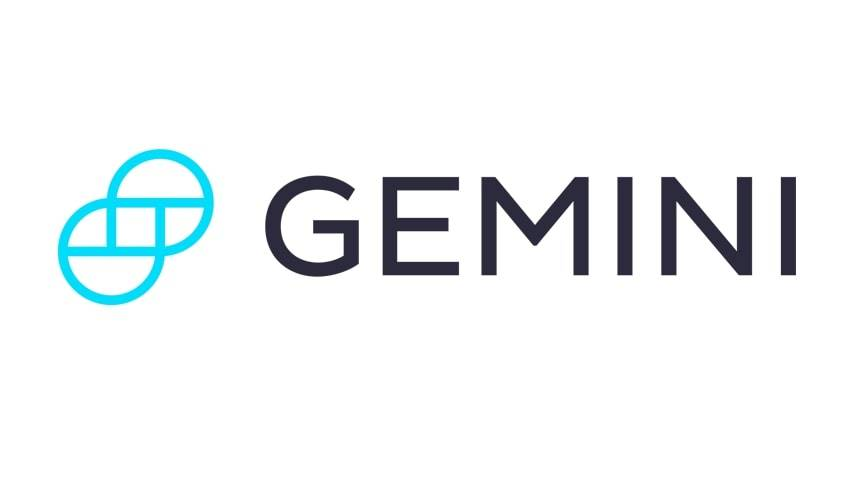# Cap RateCap rate, short for capitalization rate, is a measure of the ratio between the cash flow produced by an asset, typically real estate, and it’s capital cost. For …Cap rate, short for capitalization rate, is a measure of the ratio between the cash flow produced by an asset, typically real estate, and it’s capital cost. For simplication purposes it can be looked at as the percentage of annual return you earn on invested capital. Thus a 10% cap rate on a \$1,000,000 building will return \$100,000 per year in net cash flow. Net cash flow is the return after all expenses have been paid.

Cap rate is commonly used to measure how fast an asset will pay for itself in net cash flows.

Cap Rate = Annual Cash Flow/Capital Cost### Try Gemini Today! 123

The Gemini Exchange makes it simple to research crypto market, buy bitcoin and other cryptos plus earn Up to 8.05% APY!

For example, if a home is purchased for 800,000 and produces 200,000 in cash flow, subtracting costs, over the course of a year:

Capitalization Rate = 200,000/800,000 x 100 = 25%

Analysis of Cap Rate
A favorable cap rate in one market may be unfavorable in another market.

Using Cap Rate to Estimate Property Value
If you can estimate the annual cash flow, you can use the cap rate to estimate the property value. You can estimate the cap rate in your area by finding the cap rate used by investors for similar properties in the same market.

For instance if you determine recent cap rates used were about 25% then divide your estimated cash flow by .25 to calculate an estimated property value.

200,000 (estimated annual cash flow)/.25 (cap rate) = 800,000 (estimated property value).